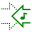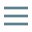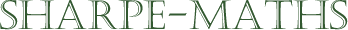# Glossary of terms

## C

•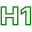### Complex numbers   ($\Bbb{C}$)

•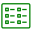Complex numbers are the outermost set of numbers. In other words, every single number anyone can name is a complex number.

The complex numbers are a 2-dimensional representation of the most general numbers we can work with in mathematics. They seem a bit silly when you first meet them and it took quite a few centuries before they were accepted by the wider mathematical community. However, they are very useful in all sorts of modelling in design, electronics and many more.

Complex numbers are made up from a real part and an imaginary part, which are written as a simple sum, like $a + ib$ where $i = \sqrt{-1}$ and $a, b$ are real numbers.

•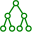•